# Collaborative filtering with embeddings

Embeddings are not just for use in natural language processing. Here we apply embeddings to a common task in collaborative filtering - predicting user ratings - and on our way, strive for a better understanding of what an embedding layer really does.

Sigrid Keydana (RStudio)https://www.rstudio.com/
09-26-2018

What’s your first association when you read the word embeddings? For most of us, the answer will probably be word embeddings, or word vectors. A quick search for recent papers on arxiv shows what else can be embedded: equations(Krstovski and Blei 2018), vehicle sensor data(Hallac et al. 2018), graphs(Ahmed et al. 2018), code(Alon et al. 2018), spatial data(Jean et al. 2018), biological entities(Zohra Smaili, Gao, and Hoehndorf 2018) … - and what not.

What is so attractive about this concept? Embeddings incorporate the concept of distributed representations, an encoding of information not at specialized locations (dedicated neurons, say), but as a pattern of activations spread out over a network. No better source to cite than Geoffrey Hinton, who played an important role in the development of the concept(Rumelhart, McClelland, and PDP Research Group 1986):

Distributed representation means a many to many relationship between two types of representation (such as concepts and neurons). Each concept is represented by many neurons. Each neuron participates in the representation of many concepts.1

The advantages are manifold. Perhaps the most famous effect of using embeddings is that we can learn and make use of semantic similarity.

Let’s take a task like sentiment analysis. Initially, what we feed the network are sequences of words, essentially encoded as factors. In this setup, all words are equidistant: Orange is as different from kiwi as it is from thunderstorm. An ensuing embedding layer then maps these representations to dense vectors of floating point numbers, which can be checked for mutual similarity via various similarity measures such as cosine distance.

We hope that when we feed these “meaningful” vectors to the next layer(s), better classification will result. In addition, we may be interested in exploring that semantic space for its own sake, or use it in multi-modal transfer learning (Frome et al. 2013).

In this post, we’d like to do two things: First, we want to show an interesting application of embeddings beyond natural language processing, namely, their use in collaborative filtering. In this, we follow ideas developed in lesson5-movielens.ipynb which is part of fast.ai’s Deep Learning for Coders class. Second, to gather more intuition, we’d like to take a look “under the hood” at how a simple embedding layer can be implemented.

So first, let’s jump into collaborative filtering. Just like the notebook that inspired us, we’ll predict movie ratings. We will use the 2016 ml-latest-small dataset from MovieLens that contains ~100000 ratings of ~9900 movies, rated by ~700 users.

## Embeddings for collaborative filtering

In collaborative filtering, we try to generate recommendations based not on elaborate knowledge about our users and not on detailed profiles of our products, but on how users and products go together. Is product $$\mathbf{p}$$ a fit for user $$\mathbf{u}$$? If so, we’ll recommend it.

Often, this is done via matrix factorization. See, for example, this nice article by the winners of the 2009 Netflix prize, introducing the why and how of matrix factorization techniques as used in collaborative filtering.

Here’s the general principle. While other techniques like non-negative matrix factorization may be more popular, this diagram of singular value decomposition (SVD) found on Facebook Research is particularly instructive.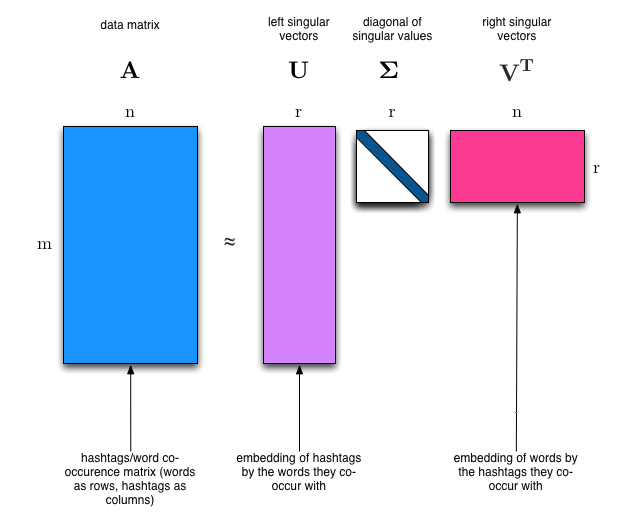Figure from https://research.fb.com/fast-randomized-svd/

The diagram takes its example from the context of text analysis, assuming a co-occurrence matrix of hashtags and users ($$\mathbf{A}$$). As stated above, we’ll instead work with a dataset of movie ratings.

Were we doing matrix factorization, we would need to somehow address the fact that not every user has rated every movie. As we’ll be using embeddings instead, we won’t have that problem. For the sake of argumentation, though, let’s assume for a moment the ratings were a matrix, not a dataframe in tidy format.

In that case, $$\mathbf{A}$$ would store the ratings, with each row containing the ratings one user gave to all movies.

This matrix then gets decomposed into three matrices:

• $$\mathbf{\Sigma}$$ stores the importance of the latent factors governing the relationship between users and movies.
• $$\mathbf{U}$$ contains information on how users score on these latent factors. It’s a representation (embedding) of users by the ratings they gave to the movies.
• $$\mathbf{V}$$ stores how movies score on these same latent factors. It’s a representation (embedding) of movies by how they got rated by said users.

As soon as we have a representation of movies as well as users in the same latent space, we can determine their mutual fit by a simple dot product $$\mathbf{m^ t}\mathbf{u}$$. Assuming the user and movie vectors have been normalized to length 1, this is equivalent to calculating the cosine similarity

$cos(\theta) = \frac{\mathbf{x^ t}\mathbf{y}}{\mathbf{||x||}\space\mathbf{||y||}}$

### What does all this have to do with embeddings?

Well, the same overall principles apply when we work with user resp. movie embeddings, instead of vectors obtained from matrix factorization. We’ll have one layer_embedding for users, one layer_embedding for movies, and a layer_lambda that calculates the dot product.

Here’s a minimal custom model that does exactly this:2


simple_dot <- function(embedding_dim,
n_users,
n_movies,
name = "simple_dot") {

keras_model_custom(name = name, function(self) {
self$user_embedding <- layer_embedding( input_dim = n_users + 1, output_dim = embedding_dim, embeddings_initializer = initializer_random_uniform(minval = 0, maxval = 0.05), name = "user_embedding" ) self$movie_embedding <-
layer_embedding(
input_dim = n_movies + 1,
output_dim = embedding_dim,
embeddings_initializer = initializer_random_uniform(minval = 0, maxval = 0.05),
name = "movie_embedding"
)
self$dot <- layer_lambda( f = function(x) { k_batch_dot(x[], x[], axes = 2) } ) function(x, mask = NULL) { users <- x[, 1] movies <- x[, 2] user_embedding <- self$user_embedding(users)
movie_embedding <- self$movie_embedding(movies) self$dot(list(user_embedding, movie_embedding))
}
})
}

We’re still missing the data though! Let’s load it. Besides the ratings themselves, we’ll also get the titles from movies.csv.


data_dir <- "ml-latest-small"
movies <- read_csv(file.path(data_dir, "movies.csv"))
ratings <- read_csv(file.path(data_dir, "ratings.csv"))

While user ids have no gaps in this sample, that’s different for movie ids. We therefore convert them to consecutive numbers, so we can later specify an adequate size for the lookup matrix.


dense_movies <- ratings %>% select(movieId) %>% distinct() %>% rowid_to_column()
ratings <- ratings %>% inner_join(dense_movies) %>% rename(movieIdDense = rowid)
ratings <- ratings %>% inner_join(movies) %>% select(userId, movieIdDense, rating, title, genres)

Let’s take a note, then, of how many users resp. movies we have.


n_movies <- ratings %>% select(movieIdDense) %>% distinct() %>% nrow()
n_users <- ratings %>% select(userId) %>% distinct() %>% nrow()

We’ll split off 20% of the data for validation. After training, probably all users will have been seen by the network, while very likely, not all movies will have occurred in the training sample.


train_indices <- sample(1:nrow(ratings), 0.8 * nrow(ratings))
train_ratings <- ratings[train_indices,]
valid_ratings <- ratings[-train_indices,]

x_train <- train_ratings %>% select(c(userId, movieIdDense)) %>% as.matrix()
y_train <- train_ratings %>% select(rating) %>% as.matrix()
x_valid <- valid_ratings %>% select(c(userId, movieIdDense)) %>% as.matrix()
y_valid <- valid_ratings %>% select(rating) %>% as.matrix()

### Training a simple dot product model

We’re ready to start the training process. Feel free to experiment with different embedding dimensionalities.


embedding_dim <- 64

model <- simple_dot(embedding_dim, n_users, n_movies)

model %>% compile(
loss = "mse",
optimizer = "adam"
)

history <- model %>% fit(
x_train,
y_train,
epochs = 10,
batch_size = 32,
validation_data = list(x_valid, y_valid),
callbacks = list(callback_early_stopping(patience = 2))
)

How well does this work? Final RMSE (the square root of the MSE loss we were using) on the validation set is around 1.08 , while popular benchmarks (e.g., of the LibRec recommender system) lie around 0.91. Also, we’re overfitting early. It looks like we need a slightly more sophisticated system.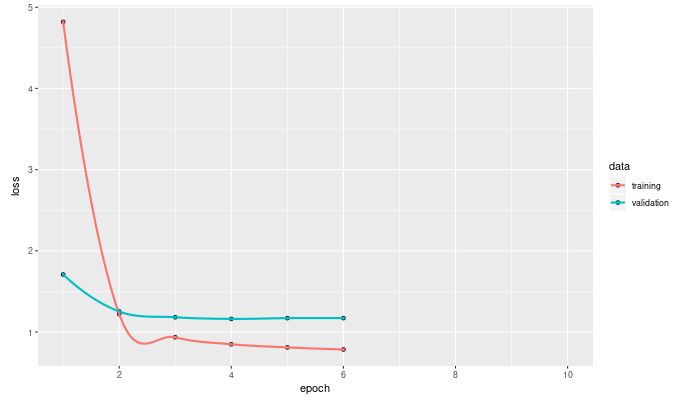Training curve for simple dot product model

### Accounting for user and movie biases

A problem with our method is that we attribute the rating as a whole to user-movie interaction. However, some users are intrinsically more critical, while others tend to be more lenient. Analogously, films differ by average rating. We hope to get better predictions when factoring in these biases.

Conceptually, we then calculate a prediction like this:

$pred = avg + bias_m + bias_u + \mathbf{m^ t}\mathbf{u}$

The corresponding Keras model gets just slightly more complex. In addition to the user and movie embeddings we’ve already been working with, the below model embeds the average user and the average movie in 1-d space. We then add both biases to the dot product encoding user-movie interaction. A sigmoid activation normalizes to a value between 0 and 1, which then gets mapped back to the original space.

Note how in this model, we also use dropout on the user and movie embeddings (again, the best dropout rate is open to experimentation).


max_rating <- ratings %>% summarise(max_rating = max(rating)) %>% pull()
min_rating <- ratings %>% summarise(min_rating = min(rating)) %>% pull()

dot_with_bias <- function(embedding_dim,
n_users,
n_movies,
max_rating,
min_rating,
name = "dot_with_bias"
) {
keras_model_custom(name = name, function(self) {

self$user_embedding <- layer_embedding(input_dim = n_users + 1, output_dim = embedding_dim, name = "user_embedding") self$movie_embedding <-
layer_embedding(input_dim = n_movies + 1,
output_dim = embedding_dim,
name = "movie_embedding")
self$user_bias <- layer_embedding(input_dim = n_users + 1, output_dim = 1, name = "user_bias") self$movie_bias <-
layer_embedding(input_dim = n_movies + 1,
output_dim = 1,
name = "movie_bias")
self$user_dropout <- layer_dropout(rate = 0.3) self$movie_dropout <- layer_dropout(rate = 0.6)
self$dot <- layer_lambda( f = function(x) k_batch_dot(x[], x[], axes = 2), name = "dot" ) self$dot_bias <-
layer_lambda(
f = function(x)
k_sigmoid(x[] + x[] + x[]),
name = "dot_bias"
)
self$pred <- layer_lambda( f = function(x) x * (self$max_rating - self$min_rating) + self$min_rating,
name = "pred"
)
self$max_rating <- max_rating self$min_rating <- min_rating

function(x, mask = NULL) {

users <- x[, 1]
movies <- x[, 2]
user_embedding <-
self$user_embedding(users) %>% self$user_dropout()
movie_embedding <-
self$movie_embedding(movies) %>% self$movie_dropout()
dot <- self$dot(list(user_embedding, movie_embedding)) dot_bias <- self$dot_bias(list(dot, self$user_bias(users), self$movie_bias(movies)))
self$pred(dot_bias) } }) } How well does this model perform?  model <- dot_with_bias(embedding_dim, n_users, n_movies, max_rating, min_rating) model %>% compile( loss = "mse", optimizer = "adam" ) history <- model %>% fit( x_train, y_train, epochs = 10, batch_size = 32, validation_data = list(x_valid, y_valid), callbacks = list(callback_early_stopping(patience = 2)) ) Not only does it overfit later, it actually reaches a way better RMSE of 0.88 on the validation set!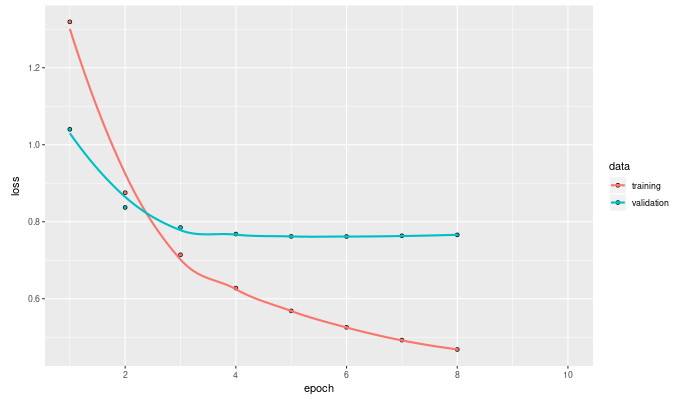Training curve for dot product model with biases Spending some time on hyperparameter optimization could very well lead to even better results. As this post focuses on the conceptual side though, we want to see what else we can do with those embeddings. ### Embeddings: a closer look We can easily extract the embedding matrices from the respective layers. Let’s do this for movies now.  movie_embeddings <- (model %>% get_layer("movie_embedding") %>% get_weights())[] How are they distributed? Here’s a heatmap of the first 20 movies. (Note how we increment the row indices by 1, because the very first row in the embedding matrix belongs to a movie id 0 which does not exist in our dataset.) We see that the embeddings look rather uniformly distributed between -0.5 and 0.5.  levelplot( t(movie_embeddings[2:21, 1:64]), xlab = "", ylab = "", scale = (list(draw = FALSE)))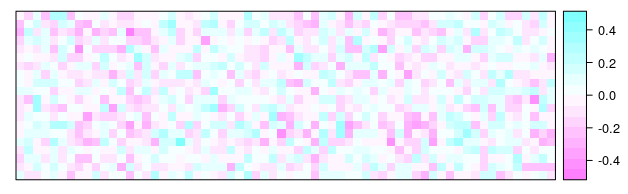Embeddings for first 20 movies Naturally, we might be interested in dimensionality reduction, and see how specific movies score on the dominant factors. A possible way to achieve this is PCA:  movie_pca <- movie_embeddings %>% prcomp(center = FALSE) components <- movie_pca$x %>% as.data.frame() %>% rowid_to_column()

plot(movie_pca)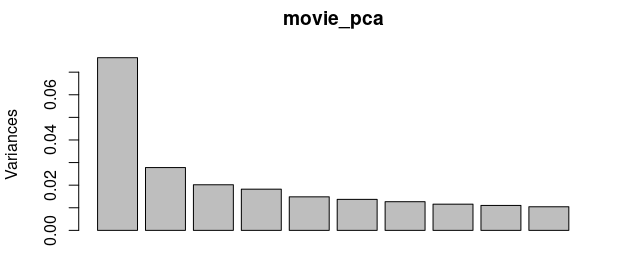PCA: Variance explained by component

Let’s just look at the first principal component as the second one already explains much less variance.

Here are the 10 movies (out of all that were rated at least 20 times) that scored lowest on the first factor:


ratings_with_pc12 <-
ratings %>% inner_join(components %>% select(rowid, PC1, PC2),
by = c("movieIdDense" = "rowid"))

ratings_grouped <-
ratings_with_pc12 %>%
group_by(title) %>%
summarize(
PC1 = max(PC1),
PC2 = max(PC2),
rating = mean(rating),
genres = max(genres),
num_ratings = n()
)

ratings_grouped %>% filter(num_ratings > 20) %>% arrange(PC1) %>% print(n = 10)

# A tibble: 1,247 x 6
title                                   PC1      PC2 rating genres                   num_ratings
<chr>                                 <dbl>    <dbl>  <dbl> <chr>                          <int>
1 Starman (1984)                       -1.15  -0.400     3.45 Adventure|Drama|Romance…          22
2 Bulworth (1998)                      -0.820  0.218     3.29 Comedy|Drama|Romance              31
3 Cable Guy, The (1996)                -0.801 -0.00333   2.55 Comedy|Thriller                   59
4 Species (1995)                       -0.772 -0.126     2.81 Horror|Sci-Fi                     55
5 Save the Last Dance (2001)           -0.765  0.0302    3.36 Drama|Romance                     21
6 Spanish Prisoner, The (1997)         -0.760  0.435     3.91 Crime|Drama|Mystery|Thr…          23
7 Sgt. Bilko (1996)                    -0.757  0.249     2.76 Comedy                            29
8 Naked Gun 2 1/2: The Smell of Fear,… -0.749  0.140     3.44 Comedy                            27
9 Swordfish (2001)                     -0.694  0.328     2.92 Action|Crime|Drama                33
10 Addams Family Values (1993)          -0.693  0.251     3.15 Children|Comedy|Fantasy           73
# ... with 1,237 more rows

And here, inversely, are those that scored highest:


ratings_grouped %>% filter(num_ratings > 20) %>% arrange(desc(PC1)) %>% print(n = 10)

A tibble: 1,247 x 6
title                                PC1        PC2 rating genres                    num_ratings
<chr>                              <dbl>      <dbl>  <dbl> <chr>                           <int>
1 Graduate, The (1967)                1.41  0.0432      4.12 Comedy|Drama|Romance               89
2 Vertigo (1958)                      1.38 -0.0000246   4.22 Drama|Mystery|Romance|Th…          69
3 Breakfast at Tiffany's (1961)       1.28  0.278       3.59 Drama|Romance                      44
4 Treasure of the Sierra Madre, The…  1.28 -0.496       4.3  Action|Adventure|Drama|W…          30
5 Boot, Das (Boat, The) (1981)        1.26  0.238       4.17 Action|Drama|War                   51
6 Flintstones, The (1994)             1.18  0.762       2.21 Children|Comedy|Fantasy            39
7 Rock, The (1996)                    1.17 -0.269       3.74 Action|Adventure|Thriller         135
8 In the Heat of the Night (1967)     1.15 -0.110       3.91 Drama|Mystery                      22
9 Quiz Show (1994)                    1.14 -0.166       3.75 Drama                              90
10 Striptease (1996)                   1.14 -0.681       2.46 Comedy|Crime                       39
# ... with 1,237 more rows

We’ll leave it to the knowledgeable reader to name these factors, and proceed to our second topic: How does an embedding layer do what it does?

## Do-it-yourself embeddings

You may have heard people say all an embedding layer did was just a lookup. Imagine you had a dataset that, in addition to continuous variables like temperature or barometric pressure, contained a categorical column characterization consisting of tags like “foggy” or “cloudy”. Say characterization had 7 possible values, encoded as a factor with levels 1-7.

Were we going to feed this variable to a non-embedding layer, layer_dense say, we’d have to take care that those numbers do not get taken for integers, thus falsely implying an interval (or at least ordered) scale. But when we use an embedding as the first layer in a Keras model, we feed in integers all the time! For example, in text classification, a sentence might get encoded as a vector padded with zeroes, like this:


2  77   4   5 122   55  1  3   0   0  

The thing that makes this work is that the embedding layer actually does perform a lookup. Below, you’ll find a very simple3 custom layer that does essentially the same thing as Keras’ layer_embedding:

• It has a weight matrix self$embeddings that maps from an input space (movies, say) to the output space of latent factors (embeddings). • When we call the layer, as in x <- k_gather(self$embeddings, x)

it looks up the passed-in row number in the weight matrix, thus retrieving an item’s distributed representation from the matrix.


SimpleEmbedding <- R6::R6Class(
"SimpleEmbedding",

inherit = KerasLayer,

public = list(
output_dim = NULL,
emb_input_dim = NULL,
embeddings = NULL,

initialize = function(emb_input_dim, output_dim) {
self$emb_input_dim <- emb_input_dim self$output_dim <- output_dim
},

build = function(input_shape) {
self$embeddings <- self$add_weight(
name = 'embeddings',
shape = list(self$emb_input_dim, self$output_dim),
initializer = initializer_random_uniform(),
trainable = TRUE
)
},

call = function(x, mask = NULL) {
x <- k_cast(x, "int32")
k_gather(self$embeddings, x) }, compute_output_shape = function(input_shape) { list(self$output_dim)
}
)
)

As usual with custom layers, we still need a wrapper that takes care of instantiation.


layer_simple_embedding <-
function(object,
emb_input_dim,
output_dim,
name = NULL,
trainable = TRUE) {
create_layer(
SimpleEmbedding,
object,
list(
emb_input_dim = as.integer(emb_input_dim),
output_dim = as.integer(output_dim),
name = name,
trainable = trainable
)
)
}

Does this work? Let’s test it on the ratings prediction task! We’ll just substitute the custom layer in the simple dot product model we started out with, and check if we get out a similar RMSE.

## Putting the custom embedding layer to test

Here’s the simple dot product model again, this time using our custom embedding layer.


simple_dot2 <- function(embedding_dim,
n_users,
n_movies,
name = "simple_dot2") {

keras_model_custom(name = name, function(self) {
self$embedding_dim <- embedding_dim self$user_embedding <-
layer_simple_embedding(
emb_input_dim = list(n_users + 1),
output_dim = embedding_dim,
name = "user_embedding"
)
self$movie_embedding <- layer_simple_embedding( emb_input_dim = list(n_movies + 1), output_dim = embedding_dim, name = "movie_embedding" ) self$dot <-
layer_lambda(
output_shape = self$embedding_dim, f = function(x) { k_batch_dot(x[], x[], axes = 2) } ) function(x, mask = NULL) { users <- x[, 1] movies <- x[, 2] user_embedding <- self$user_embedding(users)
movie_embedding <- self$movie_embedding(movies) self$dot(list(user_embedding, movie_embedding))
}
})
}

model <- simple_dot2(embedding_dim, n_users, n_movies)

model %>% compile(
loss = "mse",
optimizer = "adam"
)

history <- model %>% fit(
x_train,
y_train,
epochs = 10,
batch_size = 32,
validation_data = list(x_valid, y_valid),
callbacks = list(callback_early_stopping(patience = 2))
)

We end up with a RMSE of 1.13 on the validation set, which is not far from the 1.08 we obtained when using layer_embedding. At least, this should tell us that we successfully reproduced the approach.

## Conclusion

Our goals in this post were twofold: Shed some light on how an embedding layer can be implemented, and show how embeddings calculated by a neural network can be used as a substitute for component matrices obtained from matrix decomposition. Of course, this is not the only thing that’s fascinating about embeddings!

For example, a very practical question is how much actual predictions can be improved by using embeddings instead of one-hot vectors; another is how learned embeddings might differ depending on what task they were trained on. Last not least - how do latent factors learned via embeddings differ from those learned by an autoencoder?

In that spirit, there is no lack of topics for exploration and poking around …

Ahmed, N.K., R. Rossi, J. Boaz Lee, T.L. Willke, R. Zhou, X. Kong, and H. Eldardiry. 2018. “Learning Role-Based Graph Embeddings.” ArXiv E-Prints, February.

Alon, Uri, Meital Zilberstein, Omer Levy, and Eran Yahav. 2018. “Code2vec: Learning Distributed Representations of Code.” CoRR abs/1803.09473. http://arxiv.org/abs/1803.09473.

Frome, Andrea, Gregory S. Corrado, Jonathon Shlens, Samy Bengio, Jeffrey Dean, Marc’Aurelio Ranzato, and Tomas Mikolov. 2013. “DeViSE: A Deep Visual-Semantic Embedding Model.” In NIPS, 2121–9.

Hallac, D., S. Bhooshan, M. Chen, K. Abida, R. Sosic, and J. Leskovec. 2018. “Drive2Vec: Multiscale State-Space Embedding of Vehicular Sensor Data.” ArXiv E-Prints, June.

Jean, Neal, Sherrie Wang, Anshul Samar, George Azzari, David B. Lobell, and Stefano Ermon. 2018. “Tile2Vec: Unsupervised Representation Learning for Spatially Distributed Data.” CoRR abs/1805.02855. http://arxiv.org/abs/1805.02855.

Krstovski, K., and D.M. Blei. 2018. “Equation Embeddings.” ArXiv E-Prints, March.

Rumelhart, David E., James L. McClelland, and CORPORATE PDP Research Group, eds. 1986. Parallel Distributed Processing: Explorations in the Microstructure of Cognition, Vol. 2: Psychological and Biological Models. Cambridge, MA, USA: MIT Press.

Zohra Smaili, F., X. Gao, and R. Hoehndorf. 2018. “Onto2Vec: Joint Vector-Based Representation of Biological Entities and Their Ontology-Based Annotations.” ArXiv E-Prints, January.

1. Custom models are a recent Keras feature that allow for a flexible definition of the forward pass. While the current use case does not require using a custom model, it nicely illustrates how the network’s logic can quickly be grasped by looking at the call method.

2. It really is simple; it only works with input length = 1.

### Reuse

Text and figures are licensed under Creative Commons Attribution CC BY 4.0. The figures that have been reused from other sources don't fall under this license and can be recognized by a note in their caption: "Figure from ...".

### Citation

For attribution, please cite this work as

Keydana (2018, Sept. 26). TensorFlow for R: Collaborative filtering with embeddings. Retrieved from https://blogs.rstudio.com/tensorflow/posts/2018-09-26-embeddings-recommender/

BibTeX citation

@misc{keydana2018embeddings-recommender,
author = {Keydana, Sigrid},
title = {TensorFlow for R: Collaborative filtering with embeddings},
url = {https://blogs.rstudio.com/tensorflow/posts/2018-09-26-embeddings-recommender/},
year = {2018}
}